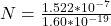## A spherical conductor with a 0.193 m radius is initially uncharged. How many electrons should be removed from the sphere in order for it to

Question

A spherical conductor with a 0.193 m radius is initially uncharged. How many electrons should be removed from the sphere in order for it to have an electrical potential of 7.10 kV at the surface?

in progress 0
5 months 2021-08-26T19:27:51+00:00 1 Answers 2 views 0

The number of electrons removed is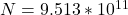Explanation:

From hr question we are told that

The radius is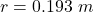The required electrical potential is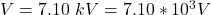The total charge on the sphere  is mathematically evaluated as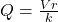where k is the coulombs constant with value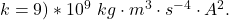substituting value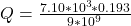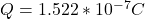The number of electron removed is mathematically evaluated as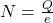Where e is  the charge on one electron with value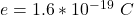substituting values